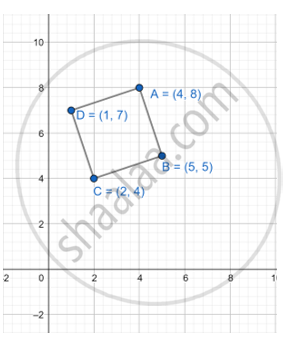# Show that Abcd is a Parallelogram If a = (4, 8), B = (5, 5), C = (2, 4), D = (1, 7) - Geometry

Sum

Show that ABCD is a parallelogram if A = (4, 8), B = (5, 5), C = (2, 4), D = (1, 7)

#### Solution

Given: A = (4, 8), B = (5, 5), C = (2, 4), D = (1, 7)

AB || DCProof:

Let A (4,8) = (x1, y1); B (5,5) = (x2, y2);

C (2,4) = (x3, y3) and D (1,7) = (x4, y4)

Distance between two points P (x1, y1) and Q (x2, y2) = ("y"_2-"y"_1)/("x"_2-"x"_1)

The slope of the line AB= ("y"_2-"y"_1)/("x"_2-"x"_1)  [Distance formula]

=(5-8)/(5-4)

=-3/1=-3  .........(i)

The slope of the line DC = ("y"_4-"y"_3)/("x"_4-"x"_3) =  [Distance formula]

=(7-4)/(1-2)

=3/(-1)=-3  ..........(ii)

The slope of the line AD=("y"_4-"y"_1)/("x"_2-"x"_1) =  [Distance formula]

= (7-4)/(1-4)

= (-1)/-3 = 1/3    ............(iii)

The slope of the line BC=("y"_3-"y"_2)/("x"_3-"x"_2) =  [Distance formula]

=(4-5)/(2-5)=(-1)/(-3) =1/3

The slope of line AB = The slope od’s the line DC [From (1) and (2)]

AB || DC

The slope of line AD = The slope of the line BC [From(3) and (4)]

Hence, ABCD is a parallelogram.

Concept: Converse: If a pair of opposite angles of a quadrilateral is supplementary, then the quadrilateral is cyclic.
Is there an error in this question or solution?